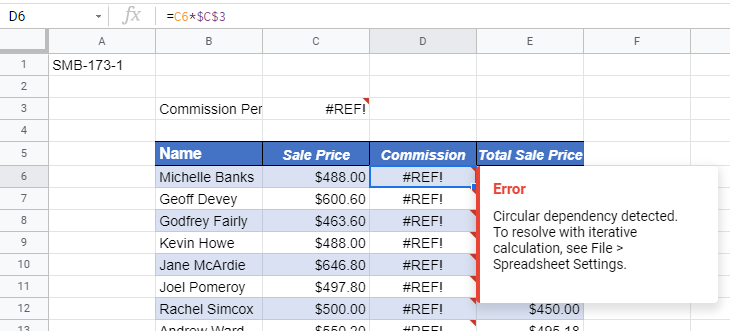# How to Find a Circular Reference Error in Excel & Google Sheets

This tutorial demonstrates how to find a circular reference error in Excel and Google Sheets.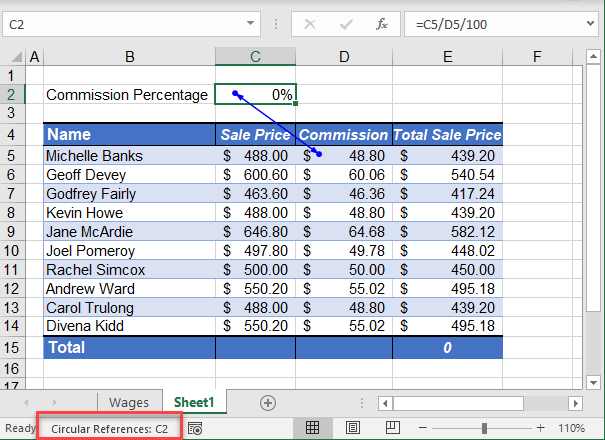There are two types of circular references in Excel: direct and indirect.

## Find Direct Circular Reference

A direct circular reference is when the formula contained in the cell refers to the cell the formula is in.

Consider the example pictured below. In the formula bar, (1) the formula =SUM(C5:C15) is stored in (2) cell C15. As C15 is the location of the formula and also part of the formula, a (3) DIRECT circular reference is found, and the formula returns a zero.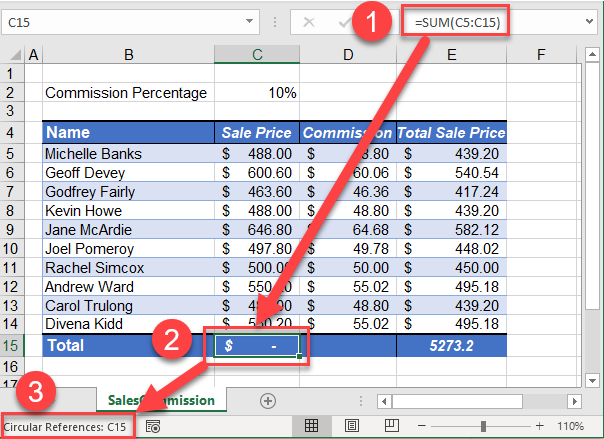1. To find instances of the error, in the Ribbon, go to Formulas > Formula Auditing > Error Checking > Circular References. The cell that contains the circular reference is shown.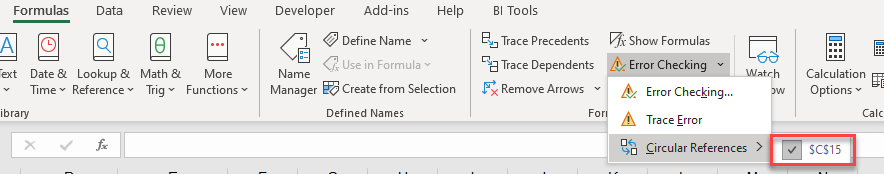1. Adjust the formula to remove the circular reference.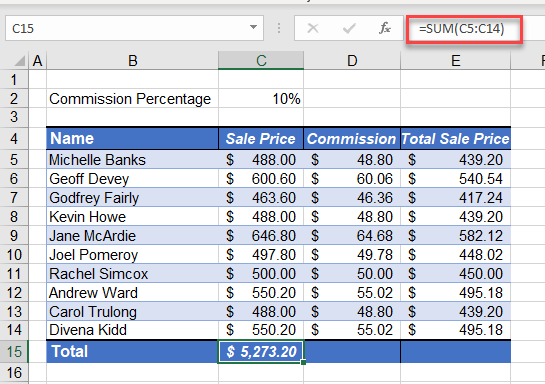## Find Indirect Circular Reference

An indirect circular reference is when a formula uses a cell that refers back to itself.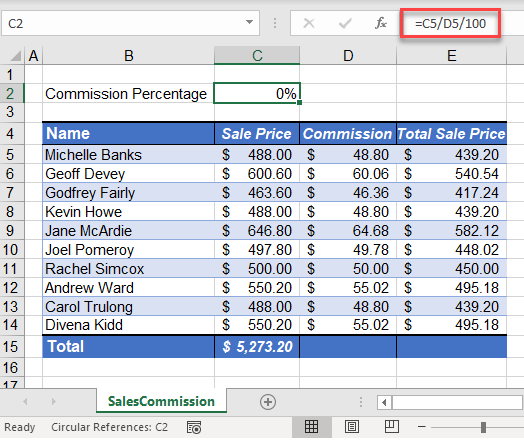The formula in Cell C2 is causing a circular reference.

1. In the Ribbon, go to Formula > Formula Auditing > Error Checking > Circular References. This shows two references.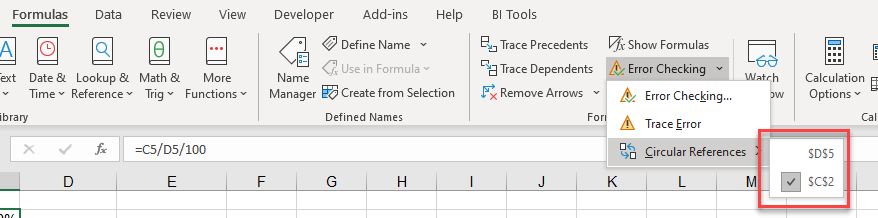1. If you select \$D\$5, the Excel pointer moves to D5 so you can examine the formula in that cell.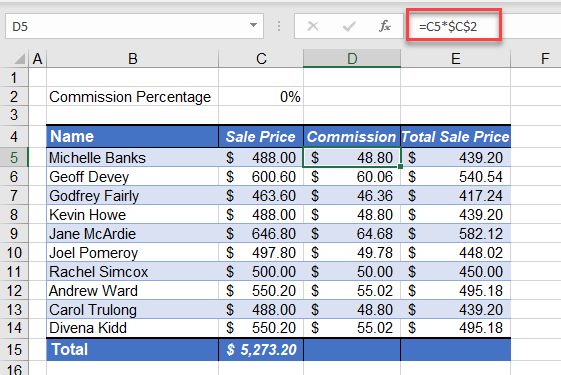If you note the formula in cell D5, it refers to cell C5, but if you note the formula in cell C5, it in turn refers to cell D5 – thus creating a circle and therefore a circular reference. These two cells cannot depend on each other. One of them needs to contain a value.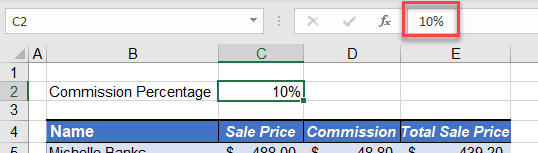1. Removing the formula in C2 and replacing it with a value removes the circular references from Excel.

Note: Another option is to enable iterative calculation and allow for circular references.

## Find Circular Reference Error in Google Sheets

Google Sheets returns an error triangle and a #REF! to any cells that contain a circular reference.